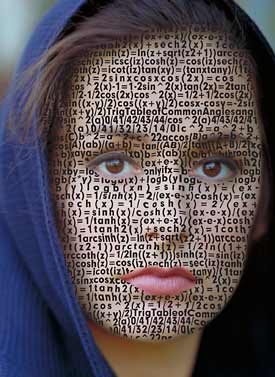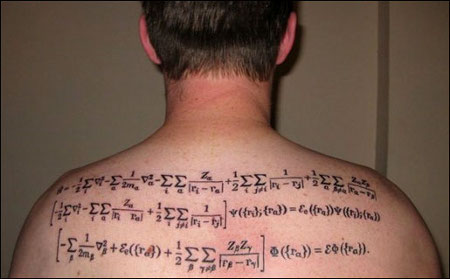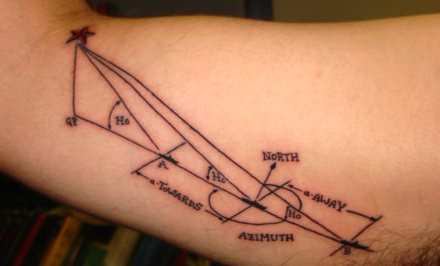Search IntMath
Close

450+ Math Lessons written by Math Professors and Teachers

5 Million+ Students Helped Each Year

1200+ Articles Written by Math Educators and Enthusiasts

Simplifying and Teaching Math for Over 23 Years

# Math body art

By Murray Bourne, 03 May 2010

Here is some trigonometric and logarithmic equations written on an attractive face (it appears to be done using Photoshop, not a tattoo. Subscripts and superscripts have been linearized.):This next guy is a biochemist and molecular biophysicist.He writes:

The first line is the Born Oppenheimer Approximation (a method for simplifying calculation of the energy and molecular wave function of a molecule), the second line is the equation in the form of a 3-Dimensional Schroedinger Equation, and the solution in the form of a Schroedinger Equation (a wave function that yields probabilities of physical variables).

And finally, a navigator in the navy believes passionately that while we have GPS, sailors should still be able to navigate using the sun and stars.The owner of the tattoo writes (original page no longer available):

For me it serves as a reminder that while technology improves, the sea remains an unpredictable place and it is up to the older generation to teach the younger the old school ways of doing business.

### 3 Comments on “Math body art”

1. Maria Droujkova says:

Angelina Jolie has tattoos of coordinates of her four children's birthplaces

2. Murray says:

Thanks, Maria. In order they appear to be Vietnam, Kenya, Namibia and Cambodia. Dedicated!

3. BEnjie says:

Wow!it's so cool having mathematical formulas on our body...

### Comment Preview

HTML: You can use simple tags like <b>, <a href="...">, etc.

To enter math, you can can either:

1. Use simple calculator-like input in the following format (surround your math in backticks, or qq on tablet or phone):
a^2 = sqrt(b^2 + c^2)
(See more on ASCIIMath syntax); or
2. Use simple LaTeX in the following format. Surround your math with $$ and $$.
$$\int g dx = \sqrt{\frac{a}{b}}$$
(This is standard simple LaTeX.)

NOTE: You can mix both types of math entry in your comment.